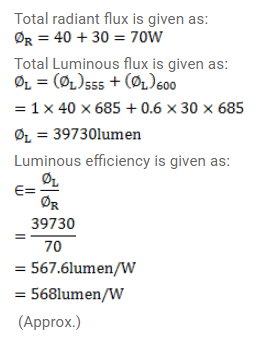# A source emits light of wavelength`
Question:

A source emits light of wavelength $555 \mathrm{~nm}$ and $600 \mathrm{~nm}$. The radiant flux of the $555 \mathrm{~nm}$ part is $49 \mathrm{~W}$ and of the $600 \mathrm{~nm}$ part is $30 \mathrm{~W}$. The relative luminosity of $600 \mathrm{~nm}$ is $0.6$. Find